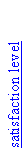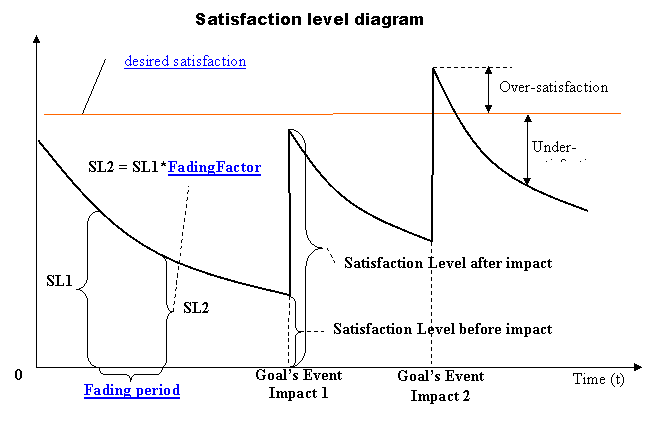# Satisfaction level diagram

The Satisfaction level diagram shows how satisfaction level of specified super goal changes with time.### Diagram elements

At the upper part of the diagram there is red line --- desired satisfaction level. It represents desirable level of satisfaction for the super goal.

Bold curve on the diagram represents how satisfaction level of the super goal is changed with time. While satisfaction level is below desired satisfaction level, the super goal wants to increase satisfaction level, because super goal is under-satisfied.

If satisfaction level is above desired satisfaction level then super goal wants to decrease satisfaction level, because the super goal is over-satisfied.

Both under-satisfaction and over-satisfaction lead to discomfort.

Discomfort level is calculated either as square of under-satisfaction or as square of over-satisfaction.

Example:

Let desired satisfaction lever = 10

Let current satisfaction level = 13 (that mean over-satisfaction for 3).

Then discomfort level = (10 – 13) ^2 = 9

### Satisfaction level curve

Let consider satisfaction level curve. On the diagram the curve consists of:

-         3 decreasing exponential curve fragments.

-         2 vertical lines.

Exponential curve fragment represents changes in satisfaction level caused by fading. The more fading factor is the more steeply satisfaction level curve is decreasing. The more Fading period is the less satisfaction level curve decreases over time. (In real PCnous implementation exponential curve fragment isn’t continuous. Actually the curve fragment looks like stairs with a step length = Fading period)

Vertical line represents momentary satisfaction level jump. This jump is caused by goal’s event impact (“goal’s event impact 1” and “goal’s event impact 2”).

### Discomfort level calculation

The closer satisfaction level to desired satisfaction level is the less reward is distributed among the cause concepts of the goal’s event impact

Note: if satisfaction level is higher than desired satisfaction level then the cause concepts will get negative reward).

Discomfort level before “Goal’s Event Impact 1” was:

discomfort level before = (desired satisfaction level – "Satisfaction Level before impact") ^ 2

Discomfort level before “Goal’s Event Impact 1” was:

discomfort level after = (desired satisfaction level – "Satisfaction Level after impact") ^ 2

### Reward calculation

So, it is easy to calculate total reward which will be distributed among the cause concepts of the “goal’s event impact 1”:

Reward = discomfort level before - discomfort level after

Example:

Let discomfort level was 15 and after the event impact discomfort level became 11. That means that Reward = 15-11 = 4. So, Reward = 4 will be distributed among concepts responsible for the impact event.